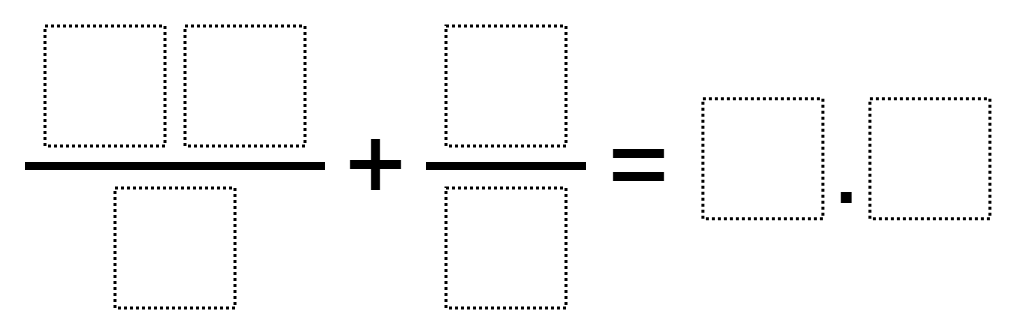# Sum of Fractions Closest to 10

Directions: Using the digits 1 through 9, at most one time each, fill in the boxes to make the statement true.### Hint

How do we add fractions? If we want to get the closest to 10, what does that mean for each of the fractions?

The closest to 10 so far is 9.8. There are few ways to get this, for example: 34/5 + 6/2 = 9.8

Source: Nanette Johnson, based on Giselle Garcia’s problem

## Equivalent Expressions with Fractions

Directions: Using the digits 0 to 9 at most one time each and choosing either …

1.I had a student come up with 9.8 as a solution:
34/5 + 6/2 = 9.8

•How??? Where should a kid start? My child needs to show the steps to solve this problem. Where do we start?

•Since the answer contains a whole number and partial number (__.__), you can plug digits into the improper fraction that would get you a whole number 9, which is as close to 10 as you can get, and then try to plug in other digits that would give you the greatest value of partial number. You have to play with it more than follow steps because of the instruction that digits can only be used once.

•Thank you for finding a 9.8 answer. The solution has been updated.

2.63/7 + 4/5=9.8
27/3+4/5=9.8

3.My student did 56/7 + 2/1 which equals 10. Does that work?

•The rule of the problems is that digits cannot be repeated. In your answer, the digit ‘1’ is used twice. Also, the answer must be a 2 digit number that has a digit in the ones place and the tenths place. 10 has a digit in the tens place and a digit in the ones place. So no, that doesn’t work.

4.I am a student in eighth grade and a solution I got was
76/8+5/9=9.944444444444444444444444444444444